Next: 6 Experiment: NbSe Up: 5 Experimental Details Previous: 5.2 The Samples

# 5.3 General Comments on the Fitting Procedure

All of the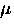SR spectra were fit in the time domain. In particular, the real and imaginary parts of the corrected asymmetry were simultaneously fit in a rotating reference frame as explained in Chapter 3. The spectra were fit with the following nine variable parameters:

1.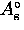: the maximum precession amplitude of the corrected asymmetry for the signal originating from within the sample.
2.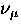: the average precession frequency of the muon spin in the sample [i.e.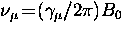,where B0 is the average internal field in Eq. (4.13)].

3.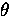: the initial phase of the muon spin polarization vector.

4.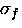: the effective depolarization rate due to random disorder in the vortex lattice and nuclear dipolar moments in the sample [see Eq. (3.24)].

5.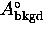: the maximum precession amplitude of the corrected asymmetry for the residual background signal (as will be explained in Chapter 6, the residual background signal was fit assuming a Gaussian broadened distribution of magnetic fields).

6.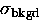: the depolarization rate corresponding to the residual background signal.

7.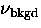: the average precession frequency of the muon spin due to the average field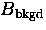of the background signal.

8.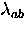: see Eq. (4.13).

9.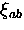: see Eq. (4.13).

The sum over reciprocal lattice vectors in Eq. (4.13) was carried out by sampling 2814 evenly spaced points in the triangle formed by three adjacent vortices. Significant changes in the fitted parameters was found for sums less than 1626 points, whereas an increase in the sum to 4902 reciprocal lattice points changed the fitted value ofby less than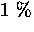.

In all cases the first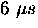of the time spectrum were fit. There was essentially no change in the fitted parameters corresponding to the sample signal when the time range was increased to.The raw data was binned so that there were approximately 1200 data points (i.e. 600 in the imaginary part and 600 in the real part of the corrected asymmetry spectrum). Doubling the bin size (to reduce the total number of data points to about 600) did not change the fitted value ofand changed the fitted value ofby no more than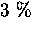in YBa2Cu3O6.95.Next: 6 Experiment: NbSe Up: 5 Experimental Details Previous: 5.2 The Samples